GeeksforGeeks App
Open AppBrowser
Continue

# Line chart in Matplotlib – Python

Matplotlib is a data visualization library in Python. The pyplot, a sublibrary of matplotlib, is a collection of functions that helps in creating a variety of charts.  Line charts are used to represent the relation between two data X and Y on a different axis. Here we will see some of the examples of a line chart in Python :

## Simple line plots

First import Matplotlib.pyplot library for plotting functions. Also, import the Numpy library as per requirement. Then define data values x and y.

## Python3

 `# importing the required libraries``import` `matplotlib.pyplot as plt``import` `numpy as np`` ` `# define data values``x ``=` `np.array([``1``, ``2``, ``3``, ``4``])  ``# X-axis points``y ``=` `x``*``2`  `# Y-axis points`` ` `plt.plot(x, y)  ``# Plot the chart``plt.show()  ``# display`

Output: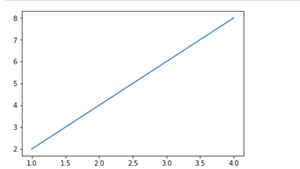Simple line plot between X and Y data

we can see in the above output image that there is no label on the x-axis and y-axis. Since labeling is necessary for understanding the chart dimensions. In the following example, we will see how to add labels, Ident in the charts

## Python3

 `import` `matplotlib.pyplot as plt``import` `numpy as np`` ` ` ` `# Define X and Y variable data``x ``=` `np.array([``1``, ``2``, ``3``, ``4``])``y ``=` `x``*``2`` ` `plt.plot(x, y)``plt.xlabel(``"X-axis"``)  ``# add X-axis label``plt.ylabel(``"Y-axis"``)  ``# add Y-axis label``plt.title(``"Any suitable title"``)  ``# add title``plt.show()`

Output: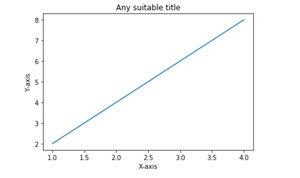Simple line plot with labels and title

## Multiple charts

We can display more than one chart in the same container by using pyplot.figure() function. This will help us in comparing the different charts and also control the look and feel of charts .

## Python3

 `import` `matplotlib.pyplot as plt``import` `numpy as np`` ` ` ` `x ``=` `np.array([``1``, ``2``, ``3``, ``4``])``y ``=` `x``*``2`` ` `plt.plot(x, y)``plt.xlabel(``"X-axis"``)``plt.ylabel(``"Y-axis"``)``plt.title(``"Any suitable title"``)``plt.show()  ``# show first chart`` ` `# The figure() function helps in creating a``# new figure that can hold a new chart in it.``plt.figure()``x1 ``=` `[``2``, ``4``, ``6``, ``8``]``y1 ``=` `[``3``, ``5``, ``7``, ``9``]``plt.plot(x1, y1, ``'-.'``)`` ` `# Show another chart with '-' dotted line``plt.show()`

Output: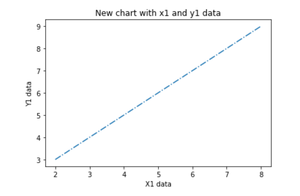## Multiple plots on the same axis

Here we will see how to add 2 plots within the same axis.

## Python3

 `import` `matplotlib.pyplot as plt``import` `numpy as np`` ` `x ``=` `np.array([``1``, ``2``, ``3``, ``4``])``y ``=` `x``*``2`` ` `# first plot with X and Y data``plt.plot(x, y)`` ` `x1 ``=` `[``2``, ``4``, ``6``, ``8``]``y1 ``=` `[``3``, ``5``, ``7``, ``9``]`` ` `# second plot with x1 and y1 data``plt.plot(x1, y1, ``'-.'``)`` ` `plt.xlabel(``"X-axis data"``)``plt.ylabel(``"Y-axis data"``)``plt.title(``'multiple plots'``)``plt.show()`

Output: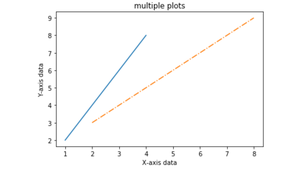## Fill the area between two plots

Using the pyplot.fill_between() function we can fill in the region between two line plots in the same graph. This will help us in understanding the margin of data between two line plots based on certain conditions.

## Python3

 `import` `matplotlib.pyplot as plt``import` `numpy as np`` ` `x ``=` `np.array([``1``, ``2``, ``3``, ``4``])``y ``=` `x``*``2`` ` `plt.plot(x, y)`` ` `x1 ``=` `[``2``, ``4``, ``6``, ``8``]``y1 ``=` `[``3``, ``5``, ``7``, ``9``]`` ` `plt.plot(x, y1, ``'-.'``)``plt.xlabel(``"X-axis data"``)``plt.ylabel(``"Y-axis data"``)``plt.title(``'multiple plots'``)`` ` `plt.fill_between(x, y, y1, color``=``'green'``, alpha``=``0.5``)``plt.show()`

Output: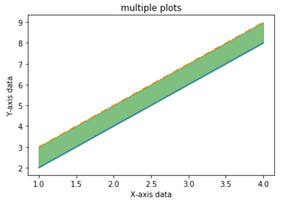Fill the area between Y and Y1 data corresponding to X-axis data

My Personal Notes arrow_drop_up